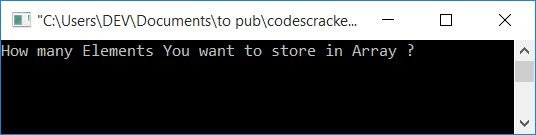# C Program for One-Dimensional Array

In this article, you will learn and get code for using a one-dimensional (1D) array in a C program. For example,

`int arr = {1, 2, 3, 4, 5, 6, 7, 8, 9, 10};`

The array arr is a one-dimensional array of size 10. Because in an array, indexing starts at 0, all the 10 numbers get stored in a way that

• The value 1 is saved at arr.
• 2 is saved at arr.
• 3 is saved at arr.
• and so on.

The last number, 10, gets stored at arr. Let's move on to the program of the one-dimensional array given below.

## One-Dimensional Array Program in C

Here is the program that uses a one-dimensional array. The question is: write a program in C that asks the user to enter the size and elements of a one-dimensional array and prints them back as output. The answer to this question is given below:

```#include<stdio.h>
#include<conio.h>
int main()
{
int arr, size, i;
printf("How many Elements You want to store in Array ? ");
scanf("%d", &size);
printf("\nEnter %d Elements: ", size);
for(i=0; i<size; i++)
scanf("%d", &arr[i]);
printf("\nElements of this One-dimensional Array is:\n");
for(i=0; i<size; i++)
printf("%d ", arr[i]);
getch();
return 0;
}```

This program was built and run under Code::Blocks. Here is the sample run:Now supply the size for a one-dimensional array, say 8, and then enter any eight numbers one by one, say 1, 2, 3, 4, 5, 6, 7, 8, and press the ENTER key to see the output as shown in the snapshot given below:#### Steps used in preceding program

These are some of the main steps used in the previous program. Supposing that the user enters 8 as the size and 1, 2, 3, 4, 5, 6, 7, and 8 as the elements of the array:

• Because the user enters 8 as the array size, 8 is set as the "size" variable.
• Initially, the value of i is 0, and 0 is less than the value of the size variable.
• So program flow goes inside the for loop, scans a value, and stores it at the index number i.
• Because the value of i is 0, the first value, 1, gets stored at str.
• Program flow increments the value of i using the third statement of the for loop, and its value becomes 1.
• Now 1 again gets checked to see if it is less than the value of size or not.
• Because 1 is again less than the value of the size variable (which holds 8 as its value),
• Then program flow again goes inside the loop and scans another value.
• This value is saved at str[i] or str.
• Now again, program flow goes to the third statement of the for loop that is the update part (of loop variable(s)) and increments the value of i.
• The value of i becomes 2, and it is checked whether it is less than the value of size or not.
• because it is less than the value of the size variable.
• So program flow again goes inside the loop.
• Continue the process until the for loop's condition evaluates to false, that is, when the value of i becomes 8, it means 8 is not less than 8 (the size value).
• So program flow exits from the first for loop.
• We have a one-dimensional array of size 8 after exiting the first for loop, with elements stored in such a way that,
• 1 is at str.
• 2 is at str.
• 3 is at str[2}.
• :
• 8 is at str.
• Now use another for loop to print the entered array back on the output screen, as shown in the program given above.

#### The same program in different languages

C Quiz

« Previous Program Next Program »